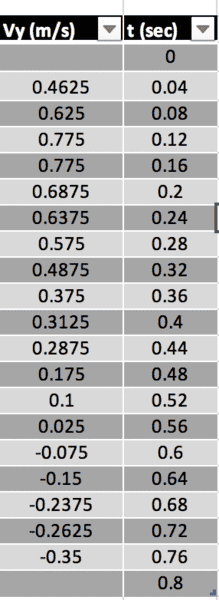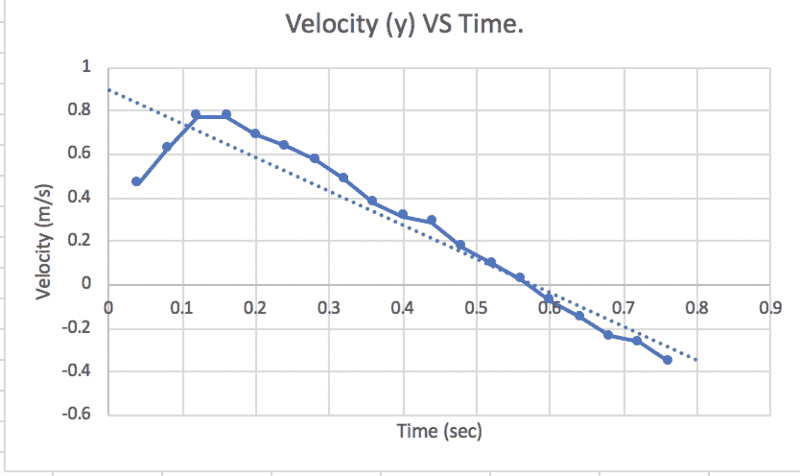# How to find the y-component of Vi based on a Vy/t graph

## Homework Statement

These are the experimental results we got from the ticker tape lab we did in projectile motion. How exactly do I find Vy? My graph looks weird. I'm assuming Vy initial must be exactly the first velocity on the table.## The Attempt at a Solution

#### Attachments

haruspex
Homework Helper
Gold Member
Please describe the experimental set up and procedure in as much detail as you can. A diagram may help.

This came from some sort of ticker tape lab, only it’s applied for projectile motion. The papers with the ticker dots were already prepared so we didn’t actually did that experiment. We measured the x and y distance for each dots, then solved for the instantaneous velocity for each time. The images I attached is the table of Vy to its corresponding time, and a graph of Vy/t

haruspex